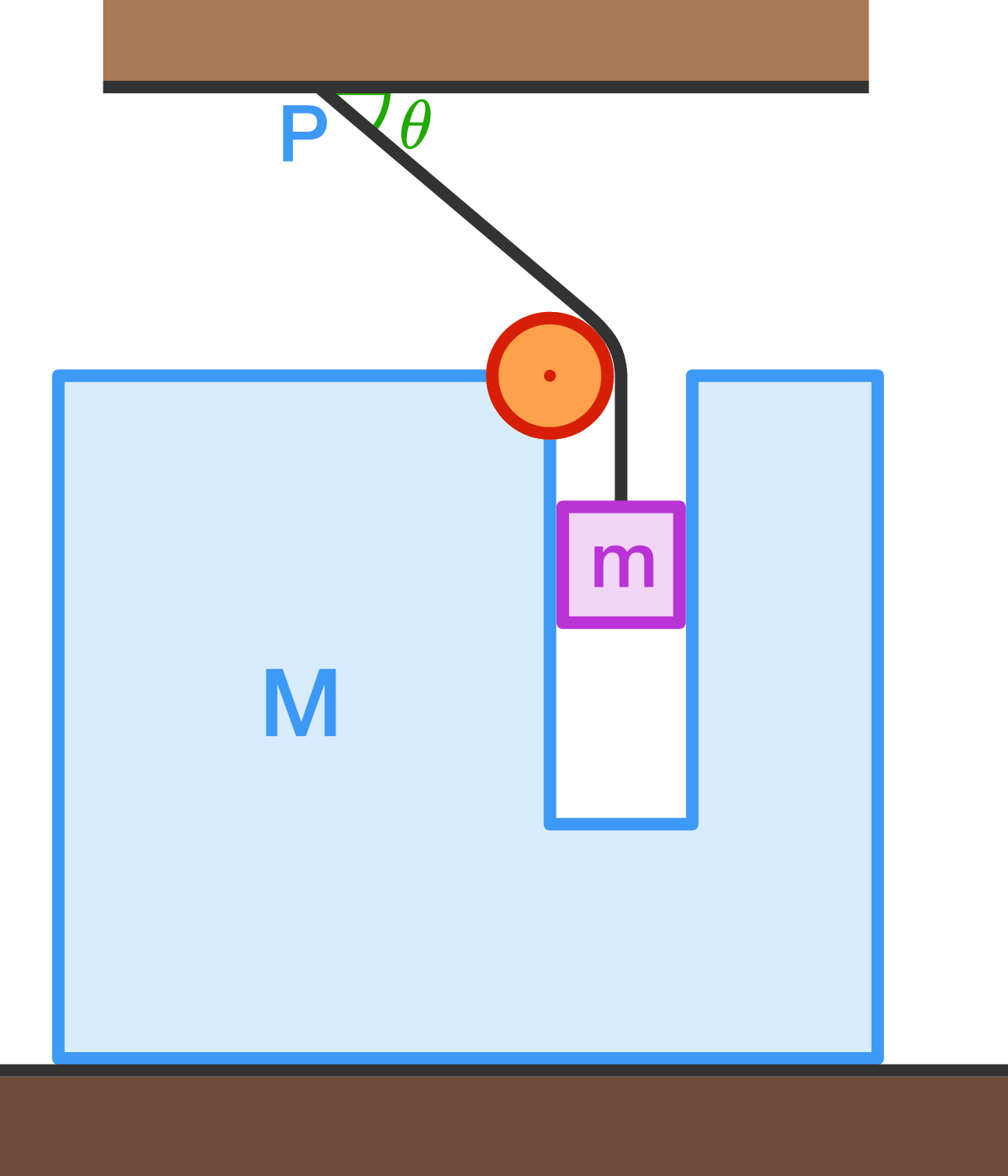# Acceleration With ConstraintA block of mass $m$ can slide freely in a slot made in a bigger block of mass $M,$ as shown in the diagram above. There is no friction anywhere in the system.

The block $m$ is connected to one end of a string whose other end is fixed at point $P$. The system is released from rest when the string at $P$ makes an angle $\theta$ with horizontal.

What is the acceleration of the smaller block with respect to the bigger block, as soon as the system is released?

Details and Assumptions:

• $m= \dfrac{1}{3} \text{ kg}$
• $M= \dfrac{1}{2} \text{ kg}$
• $\theta = 45^\circ$
• $g=10 \text{ ms}^{-2}$
×